Tip: Other languages are Google-Translated. You can visit the English version of this link.orRegisteror

## How to add number of business/working days or hours to a date in Excel?

You may need to add a number of business days or hours to a date in order to know the exact time you will finish the task during the working time. In this article, we will show you methods of adding number of business/working days or hours to a date in Excel.

### Easily add number of dates / hours / minutes to date in Excel:

Kutools for Excel's Add Dates / Hours / Minutes formulas can help you quickly add number of dates, hours or minutes to a date in Excel. See screenshot:

Kutools for Excel includes more than 300 handy Excel tools. Free to try with no limitation in 60 days. Download the free trial now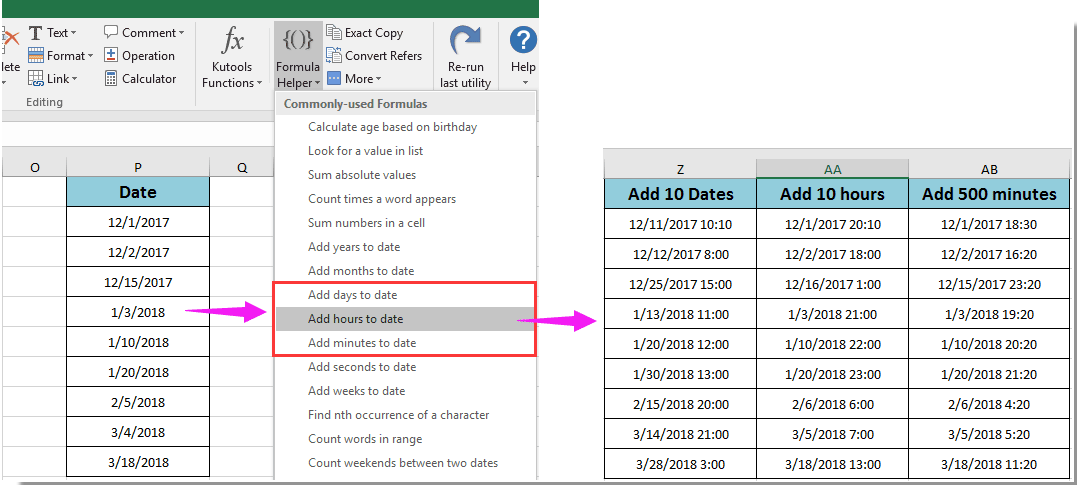Office Tab Enable Tabbed Editing and Browsing in Office, and Make Your Work Much Easier...
Kutools for Excel Solves Most of Your Problems, and Increases Your Productivity by 80%
• Reuse Anything: Add the most used or complex formulas, charts and anything else to your favorites, and quickly reuse them in the future.
• More than 20 text features: Extract Number from Text String; Extract or Remove Part of Texts; Convert Numbers and Currencies to English Words.
• Merge Tools: Multiple Workbooks and Sheets into One; Merge Multiple Cells/Rows/Columns Without Losing Data; Merge Duplicate Rows and Sum.
• Split Tools: Split Data into Multiple Sheets Based on Value; One Workbook to Multiple Excel, PDF or CSV Files; One Column to Multiple Columns.
• Paste Skipping Hidden/Filtered Rows; Count And Sum by Background Color; Send Personalized Emails to Multiple Recipients in Bulk.
• Super Filter: Create advanced filter schemes and apply to any sheets; Sort by week, day, frequency and more; Filter by bold, formulas, comment...
• More than 300 powerful features; Works with Office 2007-2019 and 365; Supports all languages; Easy deploying in your enterprise or organization.###### Save 50% of your time, and reduce thousands of mouse clicks for you every day!

Supposing date 2016/1/5 locates on cell A2, if you want to add 12 days only including working days to it without weekends, please do as follows.

1. Select a blank cell, enter formula =WORKDAY(A2,12) into the Formula Bar, and then press the Enter key. See screenshot: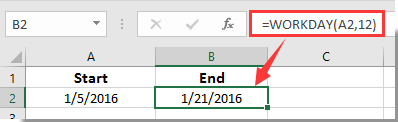Then you will get the date after adding 12 working days.

Notes:

1. In the formula, A2 is the cell contains the date you want to add working days to, 12 is the number of working days you will add to the date. Please change them as you need.

2. With the above formula, you will get the result excluding weekends. But it may include some holidays after calculating. If you want to exclude both weekends and holidays, please apply this formula =WORKDAY(A2,B2,C2).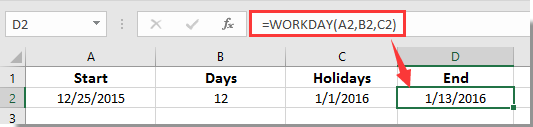In this formula, A2 contains the date you will add working days to, B2 contains the number of working days, and C2 locates the date of holiday.

3. After applying the formulas, if you get a 5-digits number, please convert the cell format to date format.

Supposing you have a start date and time, number of working hours needed to add, the start and end times of your work hours and the holiday you want to exclude as below screenshot shown. For adding number of business hours to the date, please do as follows.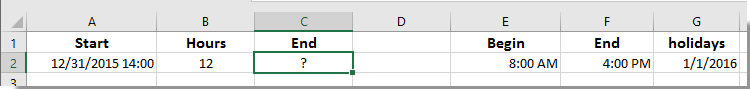1. Select a blank cell (says cell C2), enter the following formula into the formula bar, and then press the Enter key.

 =WORKDAY(A2,INT(B2/8)+IF(TIME(HOUR(A2),MINUTE(A2),SECOND(A2))+TIME(MOD(B2,8),MOD(MOD(B2,8),1)*60,0)>\$F\$2,1,0),\$G\$2:\$G\$2)+IF(TIME(HOUR(A2),MINUTE(A2),SECOND(A2))+TIME(MOD(B2,8),MOD(MOD(B2,8),1)*60,0)>\$F\$2,\$E\$2+TIME(HOUR(A2),MINUTE(A2),SECOND(A2))+TIME(MOD(B2,8),MOD(MOD(B2,8),1)*60,0)-\$F\$2,TIME(HOUR(A2),MINUTE(A2),SECOND(A2))+TIME(MOD(B2,8),MOD(MOD(B2,8),1)*60,0))

Then you can see the result as below screenshot shown.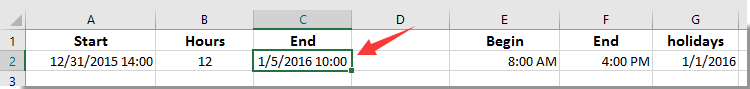Notes:

1. In the formula, A2 is the cell contains the date, B2 contains the working hours you will add to date, E2 and F2 are the start and end time of your work hours, and \$G\$2 is the specific holiday date. You can change them based on your needs.

2. If you get a number after applying this formula, please change the cell format to a date and time format.

### Kutools for Excel Solves Most of Your Problems, and Increases Your Productivity by 80%

• Reuse: Quickly insert complex formulas, charts and anything that you have used before; Encrypt Cells with password; Create Mailing List and send emails...
• Super Formula Bar (easily edit multiple lines of text and formula); Reading Layout (easily read and edit large numbers of cells); Paste to Filtered Range...
• Merge Cells/Rows/Columns without losing Data; Split Cells Content; Combine Duplicate Rows/Columns... Prevent Duplicate Cells; Compare Ranges...
• Select Duplicate or Unique Rows; Select Blank Rows (all cells are empty); Super Find and Fuzzy Find in Many Workbooks; Random Select...
• Exact Copy Multiple Cells without changing formula reference; Auto Create References to Multiple Sheets; Insert Bullets, Check Boxes and more...
• Extract Text, Add Text, Remove by Position, Remove Space; Create and Print Paging Subtotals; Convert Between Cells Content and Comments...
• Super Filter (save and apply filter schemes to other sheets); Advanced Sort by month/week/day, frequency and more; Special Filter by bold, italic...
• Combine Workbooks and WorkSheets; Merge Tables based on key columns; Split Data into Multiple Sheets; Batch Convert xls, xlsx and PDF...
• More than 300 powerful features. Supports Office/Excel 2007-2019 and 365. Supports all languages. Easy deploying in your enterprise or organization. Full features 30-day free trial.### Office Tab Brings Tabbed interface to Office, and Make Your Work Much Easier

• Enable tabbed editing and reading in Word, Excel, PowerPoint, Publisher, Access, Visio and Project.
• Open and create multiple documents in new tabs of the same window, rather than in new windows.
• Increases your productivity by 50%, and reduces hundreds of mouse clicks for you every day!Say something here...
symbols left.
###### or post as a guest, but your post won't be published automatically.
• To post as a guest, your comment is unpublished.
· 7 months ago
can some help me to get a formula for below condition
I am unable to get the time , If i do =WORKDAY.INTL(A2,B2,1) ---Output is : Deal Date: 9/11/2018 0:00, Where the time is not coming up, As time should come with 18:34 PM

A2: Deal Date :9/7/2018 18:34 PM
B2: Days: 2
Output is :New Deal Date: 9/11/2018 0:00

Required Output is 9/11/2018 18:34 PM - Plz help me with the formula!
--------------------
9/7/2018 - Friday , I need to add 2 days excluding weekends, So if i add 2 days to 9/7/2018(Friday ) its Tuesday (9/11/2018) by removing the Sat & Sun.
• To post as a guest, your comment is unpublished.
· 5 months ago
Hi Harish,
• To post as a guest, your comment is unpublished.
· 8 months ago
How to add a cell in the excel says that maximum hours per day (i.e., 8 hours) The time calculation to be bound by 8 hours per day. Please help me if we can have the spread sheet cap the calculation at 8.?
• To post as a guest, your comment is unpublished.
· 9 months ago
How to get cells in excel formula (H6; H7; H8; H9)
Forma C2 = =WORKDAY(B2;MAX(ROUNDUP((E2-IF((COUNTIF(\$J\$4:\$J\$91;INT(B2))=0)*(WEEKDAY(B2;2)<=5);IF(MOD(B2;1)>\$H\$3;0;IF(MOD(B2;1)>=\$H\$5;(H3-MOD(B2;1))*24;(\$H\$3-MAX(MOD(B2;1);\$H\$2)-(\$H\$5-MAX(MOD(B2;1);\$H\$4)))*24));0))/((\$H\$3-\$H\$2-(\$H\$5-\$H\$4))*24);0);0);\$J\$4:\$J\$91)+\$H\$2+(IFERROR(CEILING(MOD((E2-IF((COUNTIF(\$J\$4:\$J\$91;INT(B2))=0)*(WEEKDAY(B2;2)<=5);IF(MOD(B2;1)>\$H\$3;0;IF(MOD(B2;1)>=\$H\$5;(\$H\$3-MOD(B2;1))*24;(\$H\$3-MAX(MOD(B2;1);\$H\$2)-(\$H\$5-MAX(MOD(B2;1);\$H\$4)))*24));0))/((\$H\$3-\$H\$2-(\$H\$5-\$H\$4))*24);1)-0,00000001;0,00000001);1)*(\$H\$3-\$H\$2-(\$H\$5-\$H\$4))*24+(IFERROR(CEILING(MOD((E2-IF((COUNTIF(\$J\$4:\$J\$91;INT(B2))=0)*(WEEKDAY(B2;2)<=5);IF(MOD(B2;1)>H3;0;IF(MOD(B2;1)>=\$H\$5;(\$H\$3-MOD(B2;1))*24;(\$H\$3-MAX(MOD(B2;1);\$H\$2)-(\$H\$5-MAX(MOD(B2;1);\$H\$4)))*24));0))/((\$H\$3-\$H\$2-(\$H\$5-\$H\$4))*24);1)-0,00000001;0,00000001);1)*(\$H\$3-\$H\$2-(\$H\$5-\$H\$4))*24>(\$H\$4-\$H\$2)*24)*(\$H\$5-\$H\$4)*24)/24

and C3 = =WORKDAY(B3;MAX(ROUNDUP((E3-IF((COUNTIF(\$J\$4:\$J\$91;INT(B3))=0)*(WEEKDAY(B3;2)<=5);IF(MOD(B3;1)>\$H\$3;0;IF(MOD(B3;1)>=\$H\$5;(H4-MOD(B3;1))*24;(\$H\$3-MAX(MOD(B3;1);\$H\$2)-(\$H\$5-MAX(MOD(B3;1);\$H\$4)))*24));0))/((\$H\$3-\$H\$2-(\$H\$5-\$H\$4))*24);0);0);\$J\$4:\$J\$91)+\$H\$2+(IFERROR(CEILING(MOD((E3-IF((COUNTIF(\$J\$4:\$J\$91;INT(B3))=0)*(WEEKDAY(B3;2)<=5);IF(MOD(B3;1)>\$H\$3;0;IF(MOD(B3;1)>=\$H\$5;(\$H\$3-MOD(B3;1))*24;(\$H\$3-MAX(MOD(B3;1);\$H\$2)-(\$H\$5-MAX(MOD(B3;1);\$H\$4)))*24));0))/((\$H\$3-\$H\$2-(\$H\$5-\$H\$4))*24);1)-0,00000001;0,00000001);1)*(\$H\$3-\$H\$2-(\$H\$5-\$H\$4))*24+(IFERROR(CEILING(MOD((E3-IF((COUNTIF(\$J\$4:\$J\$91;INT(B3))=0)*(WEEKDAY(B3;2)<=5);IF(MOD(B3;1)>H4;0;IF(MOD(B3;1)>=\$H\$5;(\$H\$3-MOD(B3;1))*24;(\$H\$3-MAX(MOD(B3;1);\$H\$2)-(\$H\$5-MAX(MOD(B3;1);\$H\$4)))*24));0))/((\$H\$3-\$H\$2-(\$H\$5-\$H\$4))*24);1)-0,00000001;0,00000001);1)*(\$H\$3-\$H\$2-(\$H\$5-\$H\$4))*24>(\$H\$4-\$H\$2)*24)*(\$H\$5-\$H\$4)*24)/24
But the correct value is in D2 and D3. How to get it?
• To post as a guest, your comment is unpublished.
· 1 years ago
IF WE HAVE TO WRITE THE SAME FORMULA WITH WORKDAY.INTL THAN HOW TO WRITE IT
• To post as a guest, your comment is unpublished.
· 1 years ago
Please, help... this formula works perfectly if start time is between the fixed start and end times, but if the start time happens to be outside the "legal work hours", it calculates wrong end date/time, because it ads "before schedule" hours and this way the calculated end time is earlier than it should be. I have a file, where the start date/time is not manually set (imported from another list) and it can be any day and any (exact) hour. What I want is that no matter what is the start date/time, excel would still add only net work hours that are in the schedule (in my case 9:00 - 17:00).
• To post as a guest, your comment is unpublished.
· 1 years ago
Dear Agnese,
Sorry can’t help with this, you can post your question to our forum: https://www.extendoffice.com/forum.html to get more Excel supports from our professional.
• To post as a guest, your comment is unpublished.
· 1 years ago
How do i do this without business hours.
In fact, i want to sum minutes to a date, and results me the date with hours and minutes.
• To post as a guest, your comment is unpublished.
· 1 years ago
Good day,
If you want to add minutes to cell such as 500 minutes, please try this formula =A1+500/1440.
• To post as a guest, your comment is unpublished.
· 1 years ago
Hi Crystal,
Thank you for the answer, i solve my problem configuraing the "begin" and the "end" with the values "00:00" and "23:59".
To get minutes between two dates.

To add the minutes i elaborate other formula:
DataCorteHora = 1/29/18 9:00 AM
Tempo Restante = minutes